SSC  >  Quantitative Aptitude - Test 4

# Quantitative Aptitude - Test 4

Test Description

## 25 Questions MCQ Test SSC CGL Tier 1 Mock Test Series | Quantitative Aptitude - Test 4

Quantitative Aptitude - Test 4 for SSC 2022 is part of SSC CGL Tier 1 Mock Test Series preparation. The Quantitative Aptitude - Test 4 questions and answers have been prepared according to the SSC exam syllabus.The Quantitative Aptitude - Test 4 MCQs are made for SSC 2022 Exam. Find important definitions, questions, notes, meanings, examples, exercises, MCQs and online tests for Quantitative Aptitude - Test 4 below.
Solutions of Quantitative Aptitude - Test 4 questions in English are available as part of our SSC CGL Tier 1 Mock Test Series for SSC & Quantitative Aptitude - Test 4 solutions in Hindi for SSC CGL Tier 1 Mock Test Series course. Download more important topics, notes, lectures and mock test series for SSC Exam by signing up for free. Attempt Quantitative Aptitude - Test 4 | 25 questions in 15 minutes | Mock test for SSC preparation | Free important questions MCQ to study SSC CGL Tier 1 Mock Test Series for SSC Exam | Download free PDF with solutions
 1 Crore+ students have signed up on EduRev. Have you?
Quantitative Aptitude - Test 4 - Question 1

### The length of a rectangle is 2 cm more than its breadth. The diagonal is 5 cm. Find the length of the rectangle?

Detailed Solution for Quantitative Aptitude - Test 4 - Question 1

From given data
Length of the rectangle l = (b + 2)
We know that diagonal d of a rectangle = √(l2 + b2)
⇒ d = √[(b + 2)2 + b2]
⇒ 5 = √[(b + 2)2 + b2]
On squaring on both sides, we get
⇒ 25 = 2b2 + 4b + 4
⇒ 21 = 2b2 + 4b
⇒ b = -1 ± √(23/2)
∴ Length = (b + 2) = -1 ± √(23/2) + 2 = 1 ± √(23/2)

Quantitative Aptitude - Test 4 - Question 2

### The wheel of a railway carriage is 3 m in diameter and makes 8 revolutions per second, how fast is the train going?a. 27

Detailed Solution for Quantitative Aptitude - Test 4 - Question 2

From given data
Radius of the wheel r = 3/2 = 1.5 m
⇒ Circumference of the wheel = 2πr = 2π × 1. 5 = 3π = 9. 42 m
Given that wheel makes 8 revolutions per second
⇒ Distance travelled in 1 second = 8 × 9.42 = 75.36 m
⇒ Distance travelled in one hour = 75.36 × 3600 = 271296 m = 271.296 km
∴ Speed of the train ≈ 271.30 km/hr

Quantitative Aptitude - Test 4 - Question 3

### A wholesaler marks his goods 35% above the cost price. If he allows a discount of 6 1/5%, then his profit percent is

Detailed Solution for Quantitative Aptitude - Test 4 - Question 3

From given data
Let the cost price of the article be Rs. 100, then marked price = Rs. 135
Selling price = 135 – 31/5% of 135 = 135 – 8.37 = 126.63
∴ Profit percent = (126.63 – 100)/100 × 100 = 26.63 %

Quantitative Aptitude - Test 4 - Question 4

A sum of Rs. 25220 is borrowed at 5% per annum compounded annually which is paid back in 3 equal instalments. What is the amount of each instalment?

Detailed Solution for Quantitative Aptitude - Test 4 - Question 4

Let each instalment is x.
We know that Amount = p(1 + r/100)n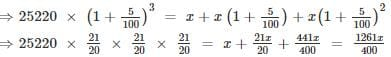⇒ x = 9261
∴ Amount at each instalment = Rs. 9261

Quantitative Aptitude - Test 4 - Question 5

The average monthly income of A and B is Rs. 5000. The average monthly income of B and C is Rs. 7250 and the average monthly income of A and C is Rs. 8500. Find the monthly income of A ?

Detailed Solution for Quantitative Aptitude - Test 4 - Question 5

From given data
Total income earned by A and B (A + B) = 2 × 5000 = Rs. 10000  …equation 1
Total income earned by B and C (B + C) = 2 × 7250 = Rs. 14500  … equation 2
Total income earned by A and C (A + C) = 2 × 8500 = Rs. 17000  … equation 3
By adding the three equations, we get
⇒ 2(A + B + C) = 41500
⇒ A + B + C = 20750  … equation 4
Now subtract eq 2 from equation 4, we get
⇒ A = 20750 – 14500 = 6250
∴ Monthly income of A = Rs. 6250

Quantitative Aptitude - Test 4 - Question 6

An good is sold at a certain price. By selling it at ¾ of that price one loses 20%. Find the profit percent at its original price.

Detailed Solution for Quantitative Aptitude - Test 4 - Question 6

From given data
Let the original selling price of article be Rs. x. Then, new selling price = Rs. ¾ x
Given that loss = 20%
∴ Cost price = (100/80 × 3x/4) = 15x/16
⇒ Profit = (x – 15x/16) = Rs. x/16
∴ Profit % = (profit/cost price × 100 )% = (x/16 × 16/15x × 100 ) = 6.66%

Quantitative Aptitude - Test 4 - Question 7

In an examination, 30% of total students failed in Hindi, 25% failed in English and 10% failed in both. Find the percentage of those students who passed in both the subjects?

Detailed Solution for Quantitative Aptitude - Test 4 - Question 7

From given data
No of students failed in hindi n(H) = 30%
No of students failed in English n (E) = 25%
No of students failed in both n(H ∩ E) = 10%
⇒ Percentage of students who failed n(H U E) = n(H) + n(E) – n(H ∩ E)
⇒ n(H U E) = 30 + 25 - 10
⇒ n(H U E) = 45
Percentage of students who passed = 100 - (Percentage of students who failed) = 100 - 45 = 55%

Quantitative Aptitude - Test 4 - Question 8

Shiva travelled from home to temple at the rate of 35 kmph and returned at the rate of 6 kmph. If the whole journey took 4 hours 45 minutes. Find the distance between home and temple.

Detailed Solution for Quantitative Aptitude - Test 4 - Question 8

From given data
Average speed of the two journey = 2xy/(x + y) = 2(35)(6)/(35 + 6) = 10.24 kmph
Distance travelled in 4 hours 45 min i. e, 4 ¾ hrs = 10.24 × 19/4 = 48.65 km
∴ Distance between home and temple = 48. 65/2 = 24.325 km

Quantitative Aptitude - Test 4 - Question 9

Find the value of x in the following expression 2x - 3 = 1/(8x - 4)

Detailed Solution for Quantitative Aptitude - Test 4 - Question 9

From the given data
⇒ 2x - 3 = 1/(8x - 4)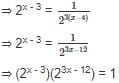When bases are equal, powers must be added
⇒ 24x - 15 = 20
On comparing on both sides, we get
⇒ 4x - 15 = 0
⇒ 4x = 15
⇒ x = 15/4

Quantitative Aptitude - Test 4 - Question 10

If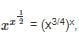then the value of x is

Detailed Solution for Quantitative Aptitude - Test 4 - Question 10

From given data
⇒ (xx)1/2 = (x3/4)x
⇒ (xx)1/2 = x3/4 x
⇒ x1/2 = ¾ x
⇒ x-1/2 = ¾
Squaring on both sides, we get
⇒ x-1 = 9/16
⇒ 1/x = 9/16
⇒ x = 16/9

Quantitative Aptitude - Test 4 - Question 11

If (4y + 72), (33 + y) are supplementary angles, find y?​

Detailed Solution for Quantitative Aptitude - Test 4 - Question 11

From given data,
We know that sum of two supplementary angles = 180°
⇒ (4y + 72) + (33 + y) = 180°
⇒ 5y + 105 = 180°
⇒ 5y = 75°
⇒ y = 15​°

Quantitative Aptitude - Test 4 - Question 12

One angle of a triangle is 60° and other angle is π/2 radian. Find the third angle in centesimal unit?

Detailed Solution for Quantitative Aptitude - Test 4 - Question 12

From the given data
∠ A = 60° and∠B = π/2 radian = 90°
We know that sum of the angles in triangle = 180°
⇒ ∠A + ∠B + ∠C = 180°
⇒ ∠C = 180 - 150 = 30°
We know that 90° = 100 grad
⇒ 30° = 30 × 100/90 = 33.33 grade

Quantitative Aptitude - Test 4 - Question 13

The value of sin 225° + sin 270° is

Detailed Solution for Quantitative Aptitude - Test 4 - Question 13

From the given data
⇒ sin (180 + 45)° + sin (270)° = -sin 45° +  (-sin 90°) = - 1/√2 – 1 = ( - 1 - √ 2 )/√ 2 = -1 - 1/√2

Quantitative Aptitude - Test 4 - Question 14

A can work twice as B and they together can finish a work in 16 days. In how many days will A alone finish the work?

Detailed Solution for Quantitative Aptitude - Test 4 - Question 14

From the given data,
⇒ Ratio of A’s work and B’s work = 2 : 1
⇒ Work done by A and B in one day = 1/16
Divide 1/16 in the ratio of 2 : 1
⇒ Work done by A in one day = (1/16 × 2/3) = 2/48 = 1/24
∴ A alone can finish the work in 24 days

Quantitative Aptitude - Test 4 - Question 15

If a + b = 26 and a - ­ b = 9 then the value of (4a2b2) is,

Detailed Solution for Quantitative Aptitude - Test 4 - Question 15

From given data
a + b = 26 and a - b = 9
By adding both the equations, we get
⇒ 2a = 35
⇒ a = 35/2
Substitute a = 35/2 in a + b = 26
⇒ 35/2 + b = 26
⇒ b = 17/2
Then value of (4a2b2) = (4 (35/2 )2(17/2)2) = 88506.25

Quantitative Aptitude - Test 4 - Question 16

If x + y = √3 and x ­- y = √2, then the value of (x2 + y2) is

Detailed Solution for Quantitative Aptitude - Test 4 - Question 16

From the given data
We know that x + y = √3 and x ­- y = √2
By adding both the equations, we get
⇒ 2x = √ 3 + √ 2
⇒ x = (√ 3 + √ 2 )/2
Substitute x = (√3 + √2)/2 in x + y = √3
⇒ y = [2√3 - (√3 + √2)]/2 = (√3 –√2 )/2
Substitute x = (√3 + √2)/2 and y = (√3 - √2)/2 in (x2 + y2)
⇒ (x2 + y2) = [{ (√3 + √2)/2 }2 + {(√3 - √2)/2 }2]
= [2(3 + 2)]/4
= 5/2

Quantitative Aptitude - Test 4 - Question 17

If the length of a chord is 24 cm which is at a certain distance from the centre of a circle of radius 13cm. Find distance between chord from centre of the circle?

Detailed Solution for Quantitative Aptitude - Test 4 - Question 17

From given data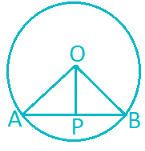Let AB = 24 cm be a chord of circle with center O and radius OA and OB equals to 13 cm
Draw OP perpendicular to AB
⇒ AP = AB/2 = 24/2 = 12 cm
From ΔOPA, we know that
⇒ OA2 = AP2 + OP2
⇒ 132 = OP2 + 122
⇒ 169 - 144 = OP2
⇒ OP = 5 cm
∴ Distance between chord from centre of the circle = OP = 5 cm

Quantitative Aptitude - Test 4 - Question 18

Each interior angle of a regular polygon is 150°. The number of sides is

Detailed Solution for Quantitative Aptitude - Test 4 - Question 18

From the given data
Each interior angle = 150°
∴ Exterior angle = 180° - 150° = 30°
⇒ Number of sides = 360°/exterior angle = 360°/30° = 12

Quantitative Aptitude - Test 4 - Question 19

If 2sin2θ + 4 cos2θ = 3, then find the value of θ ?

Detailed Solution for Quantitative Aptitude - Test 4 - Question 19

From the given data
We know that 2sin2θ + 4 cos2θ = 3
⇒ 2(1 - cos2θ ) + 4 cos2θ = 3
⇒ 2 – 2cos2θ + 4 cos2θ = 3
⇒ 2 cos2θ = 3 - 2
⇒ cos2θ = 1/2
⇒ cosθ = 1/√2
⇒ θ = 45°

Quantitative Aptitude - Test 4 - Question 20

A sum of amount at r% compound interest doubles in 3 years. In 6 years it will be k times of the original principle. What is the value of k?

Detailed Solution for Quantitative Aptitude - Test 4 - Question 20

From the given data
We know amount = p(1 + r/100 )n
In the first case
⇒ 2p = p(1 + r/100)3
⇒ 2 = (1 + r/100)3
In the second case
⇒ 22 = (1 + r/100)3]2
⇒ 4 = (1 + r/100)6
⇒ k = 4 times

Quantitative Aptitude - Test 4 - Question 21

A person standing on the bank of a river observes that the angle subtended by a tree on the opposite bank is 45°, when retreats 50 m from the bank he finds the angle to be 30°. find the height of the tree and breadth of the river ?

Detailed Solution for Quantitative Aptitude - Test 4 - Question 21

From the given data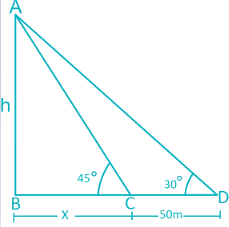Let AB be the height of the tree = h mts
Let BC be the width of the tree = x mts and CD = 50 m
⇒ ∠ ACB = 45° and ∠ADB = 30°
From ΔABC , we get
⇒ tan 45° = AB/BC
⇒ 1 = h/x
⇒ h = x m           …eq 1
From ΔABD, we get
⇒ tan 30° = AB/BD
⇒ 1/√3 = h/(x + 50)
⇒ h = (x + 50)/√3 m         …eq 2
From eq 1 and eq 2, we get
⇒ x = (x + 50)/√ 3
⇒ x(√ 3) = x + 50
⇒ x(√ 3 - 1) = 50
⇒ x = 68. 3 m
⇒ h = x = 68. 3 m
∴ Height of the tree is 68.3 m and width of the river is 68. 3 m

Quantitative Aptitude - Test 4 - Question 22

The following diagram shows the percentages of different grades of the 20000 students in the college. Observe the pie diagram and answer the questions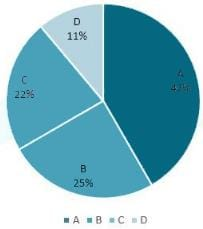Question: Find the number of students who got A grade?

Detailed Solution for Quantitative Aptitude - Test 4 - Question 22

From the given data
Percentage of number of students who got A grade = 42%
⇒ Number of students who got A grade = 42/100 × 20,000 = 8400

Quantitative Aptitude - Test 4 - Question 23

The following diagram shows the percentages of different grades of the 20000 students in the college. Observe the pie diagram and answer the questions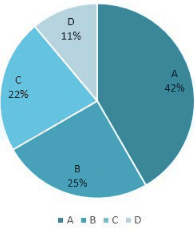Question:Find the ratio between the number of students who got A grade and B grade?

Detailed Solution for Quantitative Aptitude - Test 4 - Question 23

From the given data
Percentage of number of students who got A grade = 42%
⇒ Number of students who got A grade = 42/100 × 20,000 = 8400
Percentage of number of students who got B grade = 25%
⇒ Number of students who got B grade = 25/100 × 20,000 = 5000
∴ Ratio between the number of students who got A grade and B grade = 8400 : 5000 = 42 : 25

Quantitative Aptitude - Test 4 - Question 24

The following diagram shows the percentages of different grades of the 20000 students in the college. Observe the pie diagram and answer the questions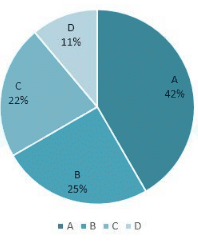Question: Find the ratio between number of students who got C grade and D grade?

Detailed Solution for Quantitative Aptitude - Test 4 - Question 24

From the given data
Percentage of number of students who got C grade = 22%
⇒ Number of students who got C grade = 22/100 × 20,000 = 4400
Percentage of number of students who got D grade = 11%
⇒ Number of students who got D grade = 11/100 × 20,000 = 2200
∴ Ratio between the number of students who got C grade and D grade = 4400 : 2200 = 2 : 1

Quantitative Aptitude - Test 4 - Question 25

The following diagram shows the percentages of different grades of the 20000 students in the college. Observe the pie diagram and answer the questionsQuestion: Find the ratio between difference of number of students who got A and B grades to the difference of the number of students who got C and D grades?

Detailed Solution for Quantitative Aptitude - Test 4 - Question 25

From the given data
Percentage of number of students who got A grade = 42%
⇒ Number of students who got A grade = 42/100 × 20, 000 = 8400
Percentage of number of students who got B grade = 25%
⇒ Number of students who got B grade = 25/100 × 20,000 = 5000
Difference of number of students who got A and B grades = 8400 – 5000 = 3400
Percentage of number of students who got C grade = 22%
⇒ Number of students who got C grade = 22/100 × 20,000 = 4400
Percentage of number of students who got D grade = 11%
⇒ Number of students who got D grade = 11/100 × 20,000 = 2200
Difference of number of students who got C and D grades = 4400 - 2200 = 2200
∴ Ratio between difference of number of students who got A and B grades to the difference of number of students who got C and D grades = 3400 : 2200 = 17 : 11

## SSC CGL Tier 1 Mock Test Series

42 docs|103 tests
 Use Code STAYHOME200 and get INR 200 additional OFF Use Coupon Code
Information about Quantitative Aptitude - Test 4 Page
In this test you can find the Exam questions for Quantitative Aptitude - Test 4 solved & explained in the simplest way possible. Besides giving Questions and answers for Quantitative Aptitude - Test 4, EduRev gives you an ample number of Online tests for practice

## SSC CGL Tier 1 Mock Test Series

42 docs|103 tests